Automation Technology
Circular Convolutional Neural Networks

# Circular Convolutional Neural Networks (CCNNs)

Schubert, S., Neubert, P., Pöschmann, J. & Protzel, P. (2019) Circular Convolutional Neural Networks for Panoramic Images and Laser Data. In Proc. of Intelligent Vehicles Symposium (IV).

Circular Convolutional Neural Networks (CCNNs) are an easy to use alternative to CNNs for input data with wrap-around structure like 360° images and multi-layer laserscans. Although circular convolutions have been used in neural networks before, a detailed description and analysis is still missing. This paper closes this gap by defining circular convolutional and circular transposed convolutional layers as the replacement of their linear counterparts, and by identifying pros and cons of applying CCNNs.

Therefore, we

• explain how circular convolution can be implemented in Circular Convolutional Layers
• derive the novel Circular Transposed Convolutional Layer that extends the application of circular convolution to a wider range of neural network architectures
• propose weight transfer to obtain a CCNN from an available, readily trained CNN
• experimentally
• evaluate properties of CCNNs using a circular MNIST classification and a Velodyne laserscanner segmentation dataset
• evaluate the performance of CCNNs transfered from pretrained CNNs (using weight transfer) without retraining
• compare CCNNs to the alternative approach input padding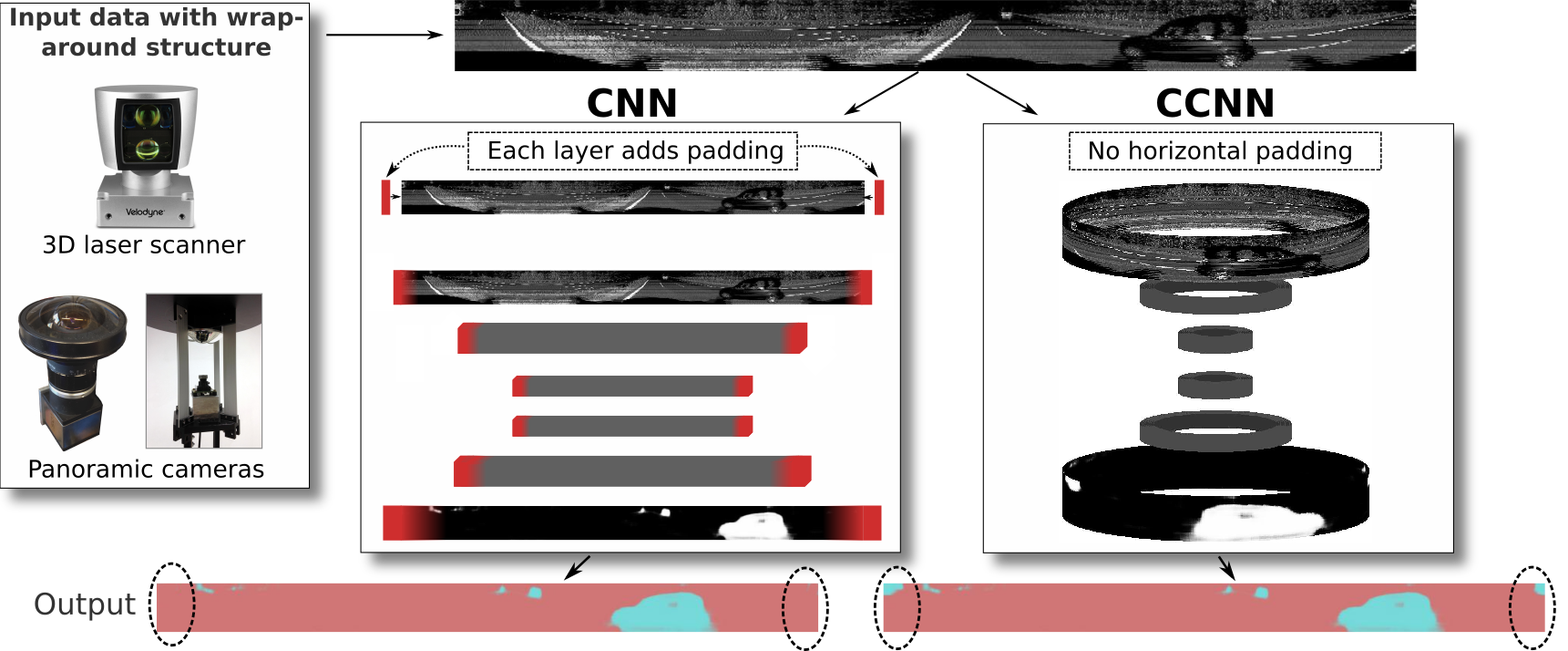## Circular convolutional layers for 1D, 2D and 3D data

In our work, we use circular (transposed) convolutional layers for 2D data like panoramic images or (projected) 3D laser data as shown below. However, the proposed circular convolutional layers can be easily extended to 1D (e.g., 2D lidar data) or 3D (e.g., grid cell networks / attractor networks).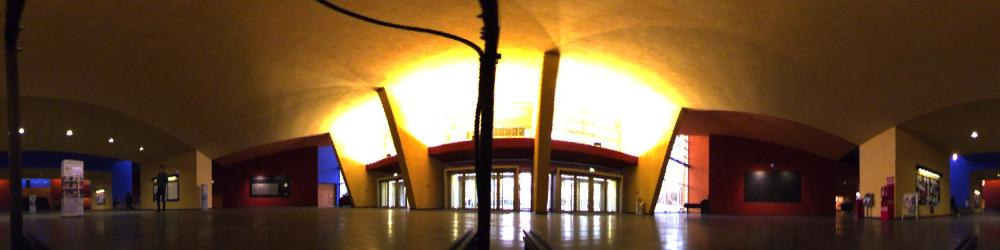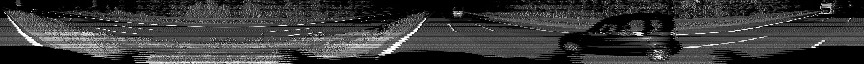## Convolutional Layer

Padding is used in convolutional layers to avoid shrinking of feature maps due to the kernel size (see animation below). This is especially important in deep neural networks that perform convolution in up to several hundred layers. In (linear) convolutional layers, this is achieved with zero-padding. In contrast, circular convolutional layers do not require zero-padding; instead the convolution kernel simply wraps around the feature map borders.

As a consequence, circular convolution prevents the involvement of zeros that propagate through the feature maps with an increasing number of layers.
## Transposed Convolutional Layer / Deconvolutional Layer

As in (linear) convolutional layers zero-padding is used in (linear) transposed convolutional layers to prevent feature maps from shrinking (see animation below). Again, this same convolution is especially important in deep neural networks. In our work, we present the novel circular transposed convolution that wraps around the image border to avoid shrinking due to the kernel size.

Like circular convolutional layers, circular transposed convolutional layers prevent the involvement of zeros from zero-padding which would propagate through the feature maps with an increasing number of layers.
## Source Code

• ccnn_layers.py
• Python3 implementation of the Circular Convolutional Layer and the Circular Transposed Convolutional Layer for Keras with Tensorflow
• Using circular convolutional layers is as easy as changing Conv2D to CConv2D or Conv2DTranspose to CConv2DTranspose in your python code with Keras.
• demo_circular_mnist_weight_transfer.py
• Demo script to compare the classification performance of a CNN and a CCNN on the circularly shifted MNIST dataset. The CNN is trained on the unshifted MNIST data. Then, the CNN-weights are copied to a CCNN (weight transfer). Finally, both networks are evaluated on all circularly shifted MNIST testing images.
• The output should be similar to the image below. We can see that the weight-transfered CCNN is independent of horizontal circular shifts.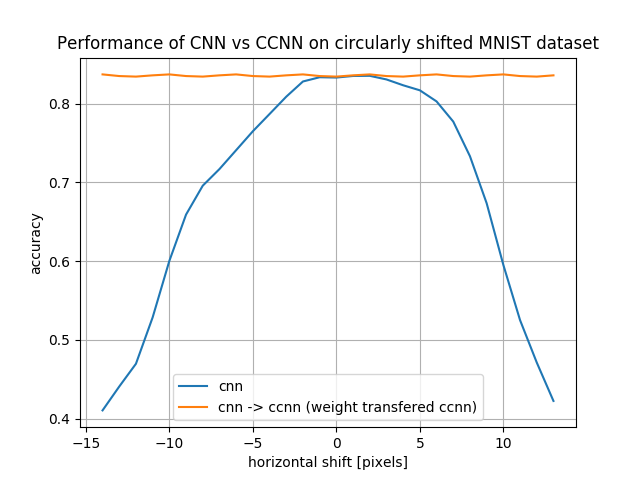## Publication

If you use circular (transposed) convolutional layers or our source code, please consider to cite the following paper:
Schubert, S., Neubert, P., Pöschmann, J. & Protzel, P. (2019) Circular Convolutional Neural Networks for Panoramic Images and Laser Data. In Proc. of Intelligent Vehicles Symposium (IV).